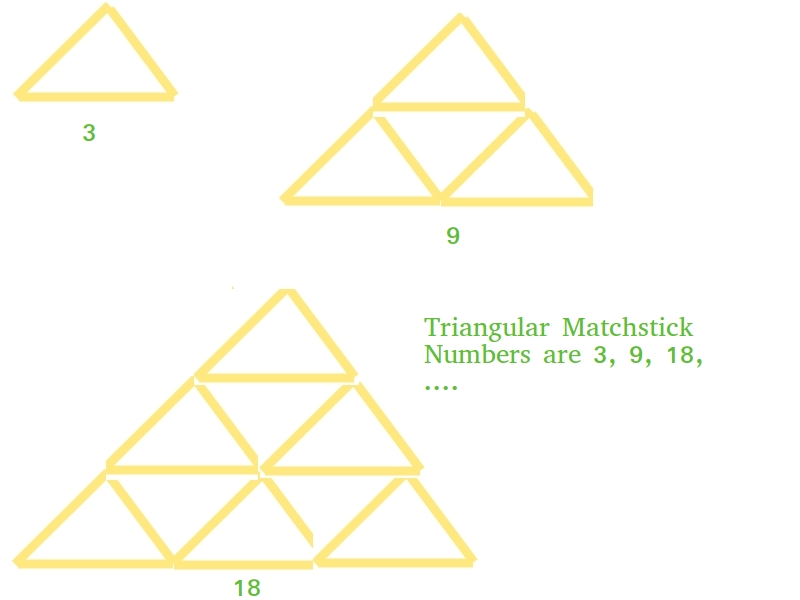Related Articles
C/C++ Program for Triangular Matchstick Number
• Last Updated : 05 Dec, 2018

Given a number X which represents the floor of a matchstick pyramid, write a program to print the total number of matchstick required to form a pyramid of matchsticks of x floors.

Examples:

```Input : X = 1
Output : 3
Input : X = 2
Output : 9
```

## Recommended: Please try your approach on {IDE} first, before moving on to the solution.

This is mainly an extension of triangular numbers. For a number X, the matchstick required will be three times of X-th triangular numbers, i.e., (3*X*(X+1))/2`// C++ program to find X-th triangular``// matchstick number`` ` `#include ``using` `namespace` `std;`` ` `int` `numberOfSticks(``int` `x)``{``    ``return` `(3 * x * (x + 1)) / 2;``}`` ` `int` `main()``{``    ``cout << numberOfSticks(7);``    ``return` `0;``}`
Output:
```84
```

Please refer complete article on Triangular Matchstick Number for more details!

Want to learn from the best curated videos and practice problems, check out the C Foundation Course for Basic to Advanced C.

My Personal Notes arrow_drop_up